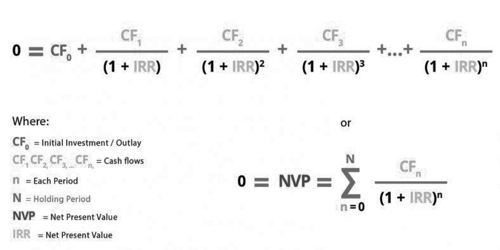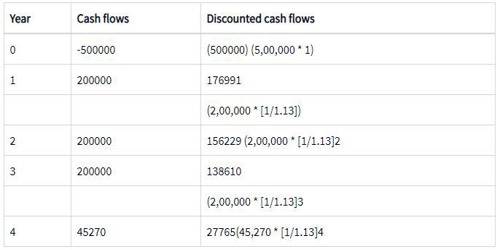The internal rate of return (IRR) is a discounting cash flow technique that gives a rate of return earned by a project. The internal rate of return is the discounting rate where the total of initial cash outlay and discounted cash inflows are equal to zero. In other words, it is the discounting rate at which the net present value (NPV) is equal to zero.

As the IRR is an estimate of a future annual rate of return, IRR should not be confused with the actually achieved investment return of historical investment. The term internal refers to the fact that the calculation excludes external factors, such as the risk-free rate, inflation, the cost of capital, or various financial risks. It is also called the discounted cash flow rate of return (DCFROR).

Formula and Calculation for IRR –

It is important for a business to look at the IRR as the plan for future growth and expansion. The formula and calculation used to determine this figure follows.

We can use the following formula to calculate IRR:

0 (NPV) = P0 + P1/(1+IRR) + P2/(1+IRR)2 + P3/(1+IRR)3 + . . . +Pn/(1+IRR)n

Where:

P0 equals the initial investment (cash outflow)

P1, P2, P3…, equals the cash flows in periods 1, 2, 3, etc.

IRR equals the project’s internal rate of return

NPV equals the Net Present Value

N equals the holding periods

ORBecause of the nature of the formula, however, IRR cannot be calculated analytically and must instead be calculated either through trial-and-error or using software programmed to calculate IRR.

Generally speaking, the higher a project’s internal rate of return, the more desirable it is to undertake. IRR is uniform for investments of varying types and, as such, IRR can be used to rank multiple prospective projects on a relatively even basis. Assuming the costs of investment are equal among the various projects, the project with the highest IRR would probably be considered the best and be undertaken first.

IRR is sometimes referred to as “economic rate of return” or “discounted cash flow rate of return.” The use of “internal” refers to the omission of external factors, such as the cost of capital or inflation, from the calculation.

The internal rate of return on an investment or project is the “annualized effective compounded return rate” or rate of return that sets the net present value of all cash flows (both positive and negative) from the investment equal to zero. Equivalently, it is the discount rate at which the net present value of the future cash flows is equal to the initial investment, and it is also the discount rate at which the total present value of costs (negative cash flows) equals the total present value of the benefits (positive cash flows).

Speaking intuitively, IRR is designed to account for the time preference of money and investments. A given return on investment received at a given time is worth more than the same return received at a later time, so the latter would yield a lower IRR than the former if all other factors are equal. A fixed income investment in which money is deposited once, interest on this deposit is paid to the investor at a specified interest rate every time period, and the original deposit neither increases nor decreases would have an IRR equal to the specified interest rate. An investment that has the same total returns as the preceding investment, but delays return for one or more time periods would have a lower IRR.

Using the IRR Formula in Excel –

A general rule of thumb is that the IRR value cannot be derived algebraically. Instead, IRR must be found by mathematical trial-and-error. This is tedious when done manually, but thankfully, Excel automatically performs this function.

3 Steps for Calculating IRR in Excel –

1. Initial Cash Flow into the Spreadsheet.

Keep in mind that this initial investment has to be a negative number. Using the example, type -300,000 into the A1 cell of the spreadsheet.

1. Subsequent Cash Flow Values for Each Period

In the cells directly under the initial investment amount, type cash flow values. For example, if our initial cash flow is in cell A1, type the following cash flows into cell A2, A3, A4, etc.

1. Instruct Excel to Calculate the IRR.

To instruct Excel to calculate IRR, type in the function command “=IRR (A1:A4)” into the A5 cell (directly under all the values).  When we hit the Enter key, the IRR value should be displayed in that cell.This same procedure can be followed for any data set, as long as the cash flow values are listed one after another in a column, directly under the initial investment amount.

If we would like to calculate IRR using our business calculator, check out these step by step IRR instructions by Investing Answers.

IRR used for capital budgeting –

If the same costs apply for different projects, then the project with the highest IRR will be selected. If an organization needs to choose between multiple investment options wherein the cost of investment remains constant, then IRR will be used to rank the projects and select the most profitable one. Ideally, the IRR higher than the cost of capital is selected.

In real-life scenarios, since the investment in any project will be huge and will have a long-term effect, an organization uses a combination of various techniques of capital budgeting like NPV, IRR, and payback period to select the best project.

Illustration – Let us say a company has an option to replace its machinery. The cost and return are as follows:

• Initial investment = Rs.5,00,000
• Incremental increase per year = Rs.2,00,000
• Replacement value = Rs.45,270
• Life of asset = 3 years

If we assume IRR to be 13%, the computation will be as follows.The total of the column Discounted Cash Flows approximately sums up to zero making the NPV equal to Zero. Hence, this discounted rate is the best rate.

As can be seen from the above, using the rate of 13%, the cash flows, both positive and negative become minimum. Hence, it is the best rate of return on investment.

The cost of capital of the company is 10%. Since the IRR is higher than the cost of capital, the project can be selected.

If the company has another opportunity to invest the money in a project that gives a 12% return, the company will still go in for the machinery replacement since it gives the highest IRR.

Information Sources: